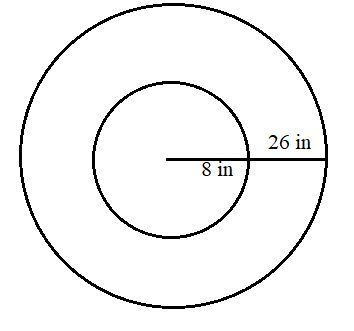# Suppose a reflector is attached to a 26 inch diameter bike wheel, 8 inches from the center. If...

## Question:

Suppose a reflector is attached to a 26 inch diameter bike wheel, 8 inches from the center.

If the bike wheel rolls without slipping, find parametric equations for the position of the reflector.

## Parametric Equation

This problem is based on the concept of parametric equations. The familiarity with the concept of parametric equations of the conic sections can be a big help. Also, while solving these types of problems it is preferable to draw picture so that we have a clearer understanding of the problem.

The picture below shows the reflector:From the figure, it is quite easy to understand that the reflector is connected 8 inches away from the center and when the wheel rotates it is quite obvious that the reflector will rotate as well in a circular motion with a radius of 8 inches.

• Therefore, it is a circle with a radius of 8 inches.

The required parametric equation can be given as,

{eq}\displaystyle \boxed{\displaystyle x=8\cos t} {/eq}

{eq}\displaystyle \boxed{\displaystyle y=8\sin t} {/eq}

Where {eq}\displaystyle t \in [0,2\pi] {/eq}.